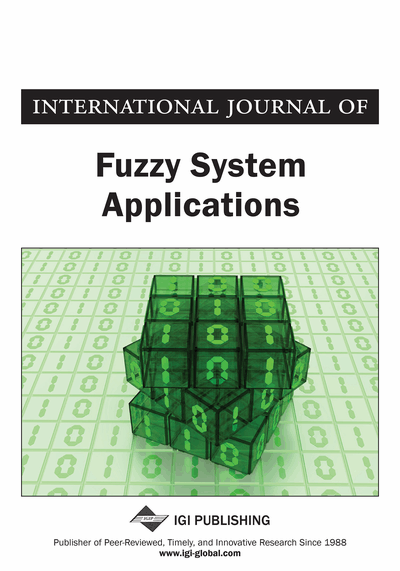# An Improved Second Order Training Algorithm for Improving the Accuracy of Fuzzy Decision Trees

Swathi Jamjala Narayanan (School of Computing Science and Engineering, VIT University, Vellore, India), Rajen B. Bhatt (Robert Bosch Research and Technology Center, Pittsburgh, PA, USA), and Ilango Paramasivam (School of Computing Science and Engineering, VIT University, Vellore, India)
DOI: 10.4018/IJFSA.2016100105
Available
\$29.50
No Current Special Offers

## Abstract

Fuzzy decision tree (FDT) is a powerful top-down, hierarchical search methodology to extract human interpretable classification rules. The performance of FDT depends on initial fuzzy partitions and other parameters like alpha-cut and leaf selection threshold. These parameters are decided either heuristically or by trial-and-error. For given set of parameters, FDT is constructed using any standard induction algorithms like Fuzzy ID3. Due to the greedy nature of induction process, there is a chance of FDT resulting in poor classification accuracy. To further improve the accuracy of FDT, in this paper, the authors propose the strategy called Improved Second Order- Neuro- Fuzzy Decision Tree (ISO-N-FDT). ISO-N-FDT tunes parameters of FDT from leaf node to roof node starting from left side of tree to its right and attains better improvement in accuracy with less number of iterations exhibiting fast convergence and powerful search ability.
Article Preview
Top

## Introduction

Machine learning algorithms like multi-layer perceptron, radial basis function (RBF) networks, neural networks and support vector machines are widely used for nonlinear pattern classification problems. In spite of having several advantages like relative ease of applications and abilities to provide gradual responses, these algorithms lack human interpretability, which can be a problem especially if users need to justify and understand their decisions. In such cases, only decision trees (DTs) managed to get satisfactory results. Decision tree is one of the most widely used classification technique due to its hierarchical representation of classification knowledge. Various decision trees are developed over the years, namely CART (Breiman et al. 1984), ID3 (Interactive Dichotomiser3), Quinlan (1986), C4.5 (Quinlan 2014), SPRINT (Shafer et al. 1996), SLIQ (Mehta et al. 1996), etc., However, crisp decision tree algorithms are criticized for their sensitivity towards the small changes in attribute values.

To address the problem related with crisp decisions, various researchers have introduced Fuzzy Decision Tree (FDT) induction algorithms (Weber, 1992; Maher and Clair, 1993; Umano et al., 1994; Yuan and Shaw, 1995; Jeng et al., 1997; Hayashi et al.,1998; Janikow, 1998; Yeung et al., 1999; Chiang & Hsu, 2002). A comprehensive survey of these FDT induction techniques can be found in Chen et al. (2009). The most important task in induction of FDT is to use an appropriate and efficient attribute selection measure. Average fuzzy classification entropy is one such measure used by Quinlan (1986) for induction of Fuzzy ID3 algorithm. Yuan and Shaw’s (1995) introduced average fuzzy classification ambiguity of attribute(s) as the measure for the induction of FDT. Both the fuzzy entropy measure and the fuzzy ambiguity measures essentially use the ratio of uncertainty to measure the significance of fuzzy conditional attributes. Further, Yeung et al. (1999) proposed the average degree of importance of attribute(s) as a novel attribute selection criterion for FDT induction. An analytic and experimental comparison of these three measures for generating FDT is given by Wang et al. (2001). Two other algorithms have been proposed by Bhatt & Gopal (2004), named as fuzzy-rough interactive dichotomizers ver. 1.1 and ver. 1.2, where they use dependency degree using fuzzy-rough hybrid method for induction of fuzzy decision tree. The description of the proposed measure is given by Bhatt & Gopal (2006). Wang & Borgelt (2004) proposed to use information gain as a splitting criterion and came up with some improvements for the same. Jensen & Shen (2005) proposed to use a fuzzy rough set based splitting criterion for FDT induction. Bhatt & Gopal (2008) proposed an attribute selection measure using fuzzy rough hybrids and produced a novel fuzzy-rough classification trees. Zhai (2011) also used fuzzy-rough technique, in which expanded attributes are selected by using significance of fuzzy conditional attributes with respect to fuzzy decision attributes. Lertworaprachaya (2014) proposed look-ahead based fuzzy decision tree induction method for constructing decision trees using interval-valued fuzzy membership values.

## Complete Article List

Search this Journal:
Reset
Volume 12: 1 Issue (2023)
Volume 11: 4 Issues (2022)
Volume 10: 4 Issues (2021)
Volume 9: 4 Issues (2020)
Volume 8: 4 Issues (2019)
Volume 7: 4 Issues (2018)
Volume 6: 4 Issues (2017)
Volume 5: 4 Issues (2016)
Volume 4: 4 Issues (2015)
Volume 3: 4 Issues (2013)
Volume 2: 4 Issues (2012)
Volume 1: 4 Issues (2011)
View Complete Journal Contents Listing